NEET  >  NCERT Exemplar: Units & Measurements - 1

# NCERT Exemplar: Units & Measurements - 1 - Notes | Study Physics Class 11 - NEET

 Table of contentsMultiple Choice QuestionsVery Short Answer Type Questions1 Crore+ students have signed up on EduRev. Have you?

Multiple Choice Questions

Q.1. The number of significant figures in 0.06900 is
(a) 5
(b) 4
(c) 2
(d) 3
Ans.
(b)
Solution.
Significant figures in the measured value of a physical quantity tell the number of digits in which we have confidence. Larger the number of significant figures obtained in a measurement, greater is the accuracy of the measurement. The reverse is also true.
The following rules are observed in counting the number of significant figures in a given measured quantity.
(i) All non-zero digits are significant.
(ii) A zero becomes significant figure if it appears between two non-zero digits.
(iii) Leading zeros or the zeros placed to the left of the number are never significant.
(iv) Trailing zeros or the zeros placed to the right of the number are significant.
(v) In exponential notation, the numerical portion gives the number of significant figures.
Leading zeros or the zeros placed to the left of the number are never.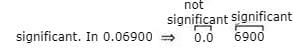Hence, number of significant figures are four.

Q.2. The sum of the numbers 436.32, 227.2 and 0.301 inappropriate significant figures is
(a) 663.821
(b) 664
(c) 663.8
(d) 663.82

Ans. (b)
Solution.
The result of an addition or subtraction in the number having different precisions should be reported to the same number of decimal places as present in the number having the least number of decimal places.
436.32
+ 227.2 ← (has only one decimal place)
+ 0.301
------------
663.821 ←(answer should be reported to one decimal place)
------------
The final result should, therefore, be rounded off to one decimal place, i.e. 664.

Q.3. The mass and volume of a body are 4.237 g and 2.5 cm3, respectively. The density of the material of the body in correct significant figures is
(a) 1.6048 g cm–3
(b) 1.69 g cm–3
(c) 1.7 g cm–3
(d) 1.695 g cm–3
Ans.
(c)
Solution.
The answer to a multiplication or division is rounded off to the same number of significant figures as possessed by the least precise term used in the calculation. The final result should retain as many significant figures as are there in the original number with the least significant figures. In the given question, density should be reported to two significant figures.
Density = 4.237g/2.5 cm3 = 1.6948
After rounding off the number, we get density = 1.7

Q.4. The numbers 2.745 and 2.735 on rounding off to 3 significant figures will give
(a) 2.75 and 2.74
(b) 2.74 and 2.73
(c) 2.75 and 2.73
(d) 2.74 and 2.74

Ans. (d)
Solution.
While rounding off measurements, we use the following rules by convention:
(i) If the digit to be dropped is less than 5, then the preceding digit is left unchanged.
(ii) If the digit to be dropped is more than 5, then the preceding digit is raised by one.
(iii) If the digit to be dropped is 5 followed by digits other than zero, then the preceding digit is raised by one.
(iv) If digit to be dropped is 5 or 5 followed by zeros, then preceding digit is left unchanged, if it is even.
(v) If digit to be dropped is 5 or 5 followed by zeros, then the preceding digit is raised by one, if it is odd.
Units and Measurements
(i) Let us round off 2.745 to 3 significant figures.
(ii) Here the digit to be dropped is 5, then preceding digit is left unchanged, if it is even.
(iii) Hence on rounding off 2.745, it would be 2.74.
(iv) Now consider 2.737, here also the digit to be dropped is 5, then the preceding digit is raised by one, if it is odd. Hence on rounding off 2.735 to 3 significant figures, it would be 2.74.

Q.5. The length and breadth of a rectangular sheet are 16.2 cm and 10.1 cm, respectively. The area of the sheet in appropriate significant figures and error is
(a) 164 ± 3 cm2
(b) 163.62 ± 2.6 cm2
(c) 163.6 ± 2.6 cm2
(d) 163.62 ± 3 cm2
Ans.
(a)
Solution.

Error in product of quantities: Suppose x = a × b
Let Δa = absolute error in measurement of a,
Δb = absolute error in measurement of b,
Δx = absolute error in calculation of x, i.e. product of a and b.
The maximum fractional error in x is Δx/x = ± (Δa/a + Δb/b)
Percentage error in the value of x = (Percentage error in value of a) + (Percentage error in value of b)
According to the problem, length l = (16.2 ± 0.1) cm
Breadth b = (10.1 ± 0.1) cm
Area A = l × b- (16.2 cm) × (10.1 cm) = 163.62 cm2
As per the rule area will have only three significant figures and error will have only one significant figure. Rounding off we get, area A = 164 cm2
If ΔA is error in the area, then relative error is calculated as ΔA/A.
ΔA/A = Δl/l + Δb/b
= 0.1 cm/16.2 cm + 0.1 cm/10.1 cm
= 1.01 + 1.62/16.2 × 10.1 = 2.63/163.62
⇒ ΔA = A × 2.63/163.62 cm2
= 163.62 × 2.63/163.62 = 2.63cm2
ΔA = 3 cm2 (By rounding off to one significant figure)
Area, A = A ± ΔA = (164 ± 3) cm2

Q.6. Which of the following pairs of physical quantities does not have same dimensional formula?
(a) Work and torque.
(b) Angular momentum and Planck’s constant.
(c) Tension and surface tension.
(d) Impulse and linear momentum.

Ans. (c)
Solution.
(a) Work = Force × displacement = [MLT-2][L]=[ML2T-2]
Torque = force × distance = [MLT-2][L]=[ML2T-2]
Both have same dimensions.
(b) Angular  momentum L = mvr = [M][LT-1][L] = [ML2T-1]
Planck's constant h = F.s/v (∴ E = hv)
= [MLT-2][L]/[T-1] = [ML2T-1]
Dimensions of h and L are equal.
(c) Tension = force = [MLT-2]
Surface tension = Force/i = [MLT-2]/[L] = [ML0T-2]
Dimensions of both are not same.
(d) Impulse = F × Δt = [MLT-2][T]=[MLT-1]
Momentum = mv = [MLT-1]
Both have same dimensions.

Q.7. Measure of two quantities along with the precision of respective measuring instrument is
A = 2.5 m s-1 ± 0.5 m s-1
B = 0.10 s ± 0.01 s

The value of AB will be
(a) (0.25 ± 0.08) m
(b) (0.25 ± 0.5) m
(c) (0.25 ± 0.05) m
(d) (0.25 ± 0.135) m

Ans. (a)
Solution.
A = (2.5 ± 0.5)ms-1
B = (0.10 ± 0.01)s
X = AB = 2.5 × 0.10 = 0.25  m
Δx/x = ΔA/A + ΔB/B
Δx/x = 0.5/2.5 + 0.01./0.10
Δx/x = 0.075/0.25, Δx = 0.007 ≅ 0.08
(Rounding off up to 2 significant figures)
∴ AB = (2.5 ± 0.08) m.

Q.8. You measure two quantities as A = 1.0 m ± 0.2 m, B = 2.0 m ± 0.2 m. We should report correct value for √AB as:
(a) 1.4 m ± 0.4 m
(b) 1.41 m ± 0.15 m
(c) 1.4 m ± 0.3 m
(d) 1.4 m ± 0.2 m

Ans. (d)
Solution.
Quantities A and b are measured quantities so, number of significant figures in 1.0 m and 2.0 m are two.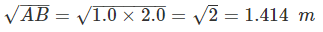rounding off up to minimum numbers of significant figure in 1.0 and 2.0 result must be in 2 significant figures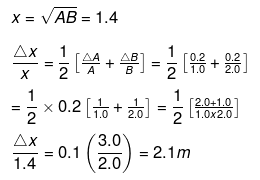Δx = 0.2 m rounding off up to 1 place of decimal.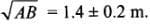Q.9. Which of the following measurements is most precise?
(a) 5.00 mm
(b) 5.00 cm
(c) 5.00 m
(d) 5.00 km

Ans. (a)
Solution.
Precision is the degree to which several measurements provide answers very close to each other. It is an indicator of the scatter in the data. The lesser the scatter, higher the precision.
Let us first check the units. In all the options magnitude is same but units of measurement are different. As here 5.00 mm has the smallest unit. All given measurements are correct up to two decimal places. However, the absolute error in (a) is 0.01 mm which is least of all the four. So it is most precise.

Q.10. The mean length of an object is 5 cm. Which of the following measurements is most accurate?
(a) 4.9 cm
(b) 4.805 cm
(c) 5.25 cm
(d) 5.4 cm

Ans. (a)
Solution.
Accuracy describes the nearness of a measurement to the standard or true value, i.e. a highly accurate measuring device will provide measurements very close to the standard, true or known values.
Example: In target shooting, a high score indicates the nearness to the bull’s eye and is a measure of the shooter’s accuracy.
According to the problem, length l = 5 cm
Let us first check the errors in each values by picking options one by one, we get
Δl1 = 5 - 4.9 = 0.1 cm,
Δl2 = 5 - 4.805 = 0.195 cm,
Δl3 = 5.25 - 5 = 0.25 cm and
Δl4 = 5.4 - 5 = 0.4 cm
Error Δl1 is the least.
Hence 4.9 cm is most closer to true value. So, 4.9 is more accurate.

Q.11. Young’s modulus of steel is 1.9 × 1011 N/m2. When expressed in CGS units of dynes/cm2, it will be equal to (1 N = 105 dyne, 1 m2 = 104 cm2)
(a) 1.9 × 1010
(b) 1.9 × 1011
(c) 1.9 × 1012
(d) 1.9 × 1013
Ans. (c)
Solution.
According to the problem.
Young's modulus, Y = 1.9 × 1011 N/m2
1 N in SI system of units = 105 dyne in C.G.S system.
Hence, 1.9 × 1011 × 105 dyne/m2
In C.G.S. length is measured in unit ‘cm’, so we should also convert m into cm.
∴ Y = 1.9 × 1011 (105dyne/104cm2) [∵ 1 m = 100 cm]
= 1.9 × 1012 dync/cm2

Q.12. If momentum (P), area (A) and time (T) are taken to be fundamental quantities, then energy has the dimensional formula
(a) (P1 A-1 T1)
(b) (P2 A1 T1)
(c) (P1 A-1/2 T1)
(d) (P1 A1/2 T-1)
Ans. (d)
Solution.
According to the problem, fundamental quantities arc momentum (p), area (A) and time (T) and we have to express energy in these fundamental quantities.
Let energy E,
E ∝ paAATc
⇒ E = kpaAATc
where, k is dimensionless constant of proportionality.
Dimensional formula of energy, [E] = [ML2 T-2] and [p] = [MLT-1]
[A] = [L2], [T] = [T] and [E] = [K][p]a[A]b[T]c
Putting all the dimensions, we get
ML2T-2 = [MLT-1]a[L2]b[T]c
= MaLa+2b T-a+c
According to the principle of homogeneity of dimensions, we get
a = 1  .... (i)
a + 2b = 2  ....(ii)
- a + c = - 2  ... (iii)
By solving these equations (i), (ii) and (iii), we get
a = 1, b = 1/2, c = -1
Dimensional formula for E is [p1 A1/2 t-1].

Q.13. On the basis of dimensions, decide which of the following relations for the displacement of a particle undergoing simple harmonic motion is not correct:
(a) y = a sin 2πt/T
(b) y = a sin vt.
(c)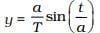(d)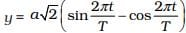Ans. (b, c)
Solution.
The argument of trigonometric functions (sin, cos etc.) should be dimensionless. y is displacement and according to the principle of homogeneity of dimensions LHS and RHS.
[Y] = [L], [a] =  [L]
[2πt/T] = [T/T]
[vt] = [v] [t] = [LT-1] [T] = [L]
[a/T]/[a]/[T] = [L]/[T] = [LT-1]
[t/a] = [LT-1]
[LHS] ≠ [RHS]
Hence, (c) is not the correct option.
⇒ LHS ≠ RHS.
So, option (b) is also not correct.

Q.14. If P, Q, R are physical quantities, having different dimensions, which of the following combinations can never be a meaningful quantity?
(a) (P – Q)/R
(b) PQ – R
(c) PQ/R
(d) (PR – Q2)/R
(e) (R + Q)/P

Ans. (a, e)
Solution.
Principle of Homogeneity of dimensions: It states that in a correct equation, the dimensions of each term added or subtracted must be same. Every correct equation must have same dimensions on both sides of the equation.
According to the problem P, Q and R are having different dimensions, since, sum and difference of physical dimensions, are meaningless, i.e., (P – Q) and (R + Q) are not meaningful.
So in option (b) and (c), PQ may have the same dimensions as those of R and in option (d) PR and Q2 may have same dimensions as those of R.
Hence, they cannot be added or subtracted, so we can say that (a) and (e) are not meaningful.

Q.15. Photon is quantum of radiation with energy E = hν where ν is frequency and h is Planck’s constant. The dimensions of h are the same as that of
(a) Linear impulse
(b) Angular impulse
(c) Linear momentum
(d) Angular momentum

Ans. (b, d)
Solution.
∴ E = hv
h = E/v = [ML2T-2]/[T-1] = [ML2T-1]
Linear impulse = F.t = dp/dt.dt = dp
= mv = [MLT-1]
Angular impulse =τ.dt = dL/dt.dt = dL = mvr
= [M][LT-1][L] = [ML2T-1]
Linear momentum = mv = [MLT-1]
Angular momentum L = mvr = [ML2T-1]
So the dimensional formulae of h, Angular impulse and Angular momentum are same.

Q.16. If Planck’s constant (h) and speed of light in vacuum (c) are taken as two fundamental quantities, which one of the following can, in addition, be taken to express length, mass and time in terms of the three chosen fundamental quantities?
(a) Mass of electron (me)
(b) Universal gravitational constant (G)
(c) Charge of electron (e)
(d) Mass of proton (mp)

Ans. (a, b, d)
Solution.
We know that dimension of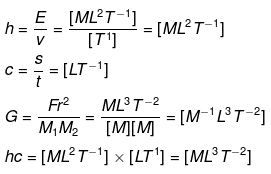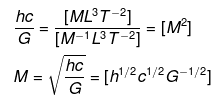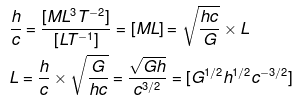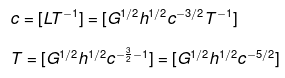Hence, physical quantities (a, b and d) can be used to represent L, M,T in terms of the choosen fundamental quantities.

Q.17. Which of the following ratios express pressure?
(a) Force/Area
(b) Energy/Volume
(c) Energy/Area
(d) Force/Volume

Ans. (a, b)
Solution.
Let us first express the relation of pressure with other physical quantities one by one with the help of dimensional analysis.
We know that pressure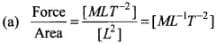So, this ratio express pressure (In fact this ratio actually represents pressure).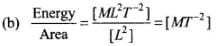Dimensions of this ratio are not same as pressure, so this ratio does not express pressure.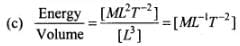Dimensions of this ratio is the same as pressure, so this ratio also express pressure.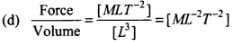Dimensions of this ratio are not same as pressure, so this ratio does not express pressure.

Q.18. Which of the following are not a unit of time?
(a) Second
(b) Parsec
(c) Year
(d) Light year

Ans. (b, d)
Solution.
Parsec and light year are those practical units which are used to measure large distances. For example, the distance between sun and earth or other celestial bodies. So they are the units of length not time. Here, second and year represent time.
Important point: 1 light year (distance that light travels in 1 year with speed = 3 × 108 m/s.) = 9.46 × 1011 m and 1 par see = 3.08 × 1016 m

Q.1. Why do we have different units for the same physical quantity?
Ans. Magnitude of any given physical quantity may vary over a wide range, therefore, different units of same physical quantity are required.
Example:
(a) Mass ranges from 10-30 kg (for an electron) to 1053 kg (for the known universe). We need different units to measure them like miligram, gram, kilogram etc.
(b) The length of a pen can be easily measured in cm, the height of a tree can be measured in metres, the distance between two cities can be measured in kilometres and distance between two heavenly bodies can be measured in light year.

Q.2. The radius of atom is of the order of 1 Å and radius of nucleus is of the order of fermi. How many magnitudes higher is the volume of atom as compared to the volume of nucleus?
Ans. Radius (R) of  atom = 1 = 10-10 m
Radius (r) of nucleus = 1 fermi =10-15 m
Ratio  of  volume  of atom to nucleus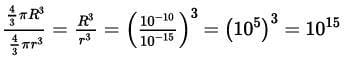Q.3. Name the device used for measuring the mass of atoms and molecules.
Ans. A mass spectrograph is a device which is used for measuring the mass of atoms and molecules.

Q.4. Express unified atomic mass unit in kg.
Ans. The unified atomic mass unit is the standard unit that is used for indicating mass on an atomic or molecular scale (atomic mass). One unified atomic mass unit is approximately the mass of one nucleon (either a single proton or neutron) and is numerically equivalent to 1 g/mol. It is defined as one- twelfth of the mass of an unbound neutral atom of carbon-12 in its nuclear and electronic ground state.

Q.5. A function f (θ) is defined as: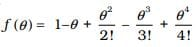Why is it necessary for f(θ) to be a dimensionless quantity?
Ans. θ is represented by angle which is equal to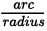so angle θ is dimensionless physical quantity.First term is 1 which is dimensionless, next term contain only powers of θ, as θ is dimensionless so their powers will also be dimensionless. Hence, each term in R.H.S. expression are dimensionless so left hand side f(θ) must be dimensionless.

Q.6. Why length, mass and time are chosen as base quantities in mechanics?
Ans. Normally each physical quantity requires a unit or standard for its specification, so it appears that there must be as many units as there are physical quantities. However, it is not so. It has been found that if in mechanics we choose arbitrarily units of any three physical quantities we can express the units of all other physical quantities in mechanics in terms of these. So, length, mass and time are chosen as base quantities in mechanics because
(a) Length, mass and time cannot be derived from one another, that is these quantities are independent.
(b) All other quantities in mechanics can be expressed in terms of length, mass and time.

The document NCERT Exemplar: Units & Measurements - 1 - Notes | Study Physics Class 11 - NEET is a part of the NEET Course Physics Class 11.
All you need of NEET at this link: NEET

## Physics Class 11

127 videos|464 docs|210 tests
 Use Code STAYHOME200 and get INR 200 additional OFF

## Physics Class 11

127 videos|464 docs|210 tests

### How to Prepare for NEET

Read our guide to prepare for NEET which is created by Toppers & the best Teachers

Track your progress, build streaks, highlight & save important lessons and more!

,

,

,

,

,

,

,

,

,

,

,

,

,

,

,

,

,

,

,

,

,

;